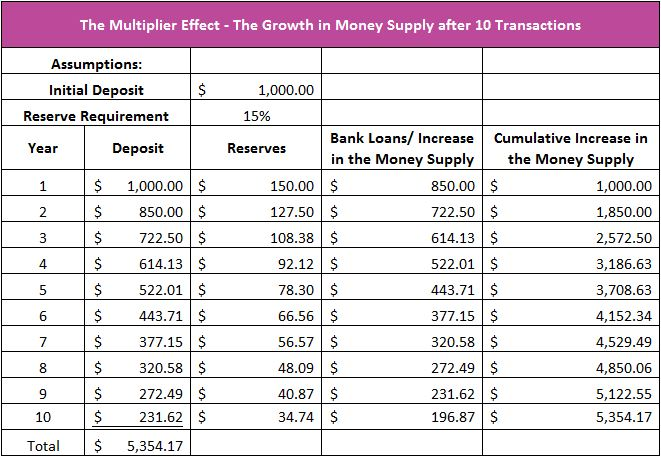# Monetary Multiplier Effect

## Definition of Monetary Multiplier Effect:

The monetary multiplier effect occurs when banks lend more than they hold in deposits and the increase in the money supply exceeds the amount of the initial deposit due to the fractional reserve banking system.

## Detailed Explanation:

An injection of money into an economy can have a ripple effect. It may be an injection of deposits, or it may be an increase in federal spending. Each creates a type of multiplier effect. The increase in fiscal spending causes a change in the economy’s aggregate demand that exceeds the amount of the initial change. This is the fiscal multiplier effect.

The monetary multiplier effect is created by fractional reserve banking. Banks add to the money supply when they lend money because they accept a deposit, retain a portion of it, and lend out the rest. To illustrate, assume Julie deposits \$1,000 in Bank A. Bank A then lends \$850 to Joe. Bank A does not give Joe cash. Instead, they write Joe a check which Joe deposits in Bank A or any other bank. Deposits have increased to \$1,850 (Julie’s initial \$1,000 deposit plus Joe’s loan proceeds of \$850). The increase in the amount deposited (\$850) increases the money supply because demand deposits are considered part of the money supply. Assume Joe deposits his \$850 in Bank B. Bank B retains reserves of \$127.50 and lends out \$722.50. The \$722.50 is deposited in another bank. This bank retains \$108.38 and lends out \$614.12. The cycle continues. The table below summarizes the growth of the money supply for ten transactions when each bank retains 15 percent of its deposits in reserve. Note that the money supply increase is less after each transaction. It does not matter which bank receives the deposited money. In each case, 15 percent is retained for reserves and 85 percent of the deposit is loaned.The reserve requirement has an indirect relationship with the money multiplier. Banks have more money to lend if less money is kept in reserve. The maximum increase or monetary multiplier can be calculated using the formula below where MM is the monetary multiplier and RR is the reserve requirement.

MM = 1 / RR

If the above formula is used we can determine that a deposit of \$1,000 could increase the money supply \$6,667.

MM = 1 / .15
MM = 6.667
\$1,000 x 6.667 = \$6,667

Realistically, not all of the cash loaned out is redeposited, and banks do not lend all of their excess reserves. Money that is not redeposited cannot contribute to the expansion of the money supply. Leakage occurs when borrowers hold currency or when banks hold excess reserves. The US Reserve requirement is ten percent, yet because of leakage, the Federal Reserve Band of St. Louis estimates the M1 multiplier is closer to one! Open the link to FRED for the current M1 multiplier and historical graph. The revised formula for the multiplier with leakage equals. LR is the leakage ratio.

MM = (1+ LR)
(RR + LR)

Assume that the central bank of Tiny Nation wants to expand its economy. It sets a target multiplier of 20. What should it set as its reserve requirement if it assumes no leakage?

20 = 1/R

Solving for RR we can determine that Tiny Nation should have a reserve requirement of five percent. Now assume that Tiny Nation’s population hordes its cash because they are fearful of a depression. People and banks retain an average 25 percent of their deposits. What is the resulting money multiplier?

MM = (1 + .25)
(.5 + .25)
MM = 4.17

## Dig Deeper With These Free Lessons:

Fractional Reserve Banking and The Creation of Money
Monetary Policy – The Power of an Interest Rate
Fiscal Policy – Managing an Economy By Taxing and Spending
Gross Domestic Product – Measuring an Economy's Performance
What is Money